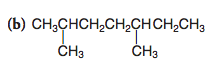# Problem: Rewrite each of the following using bond-line formulas:

🤓 Based on our data, we think this question is relevant for Professor de St Germain's class at FAU.

###### Problem Details

Rewrite each of the following using bond-line formulas:What scientific concept do you need to know in order to solve this problem?

Our tutors have indicated that to solve this problem you will need to apply the Skeletal Structure concept. You can view video lessons to learn Skeletal Structure. Or if you need more Skeletal Structure practice, you can also practice Skeletal Structure practice problems.

What is the difficulty of this problem?

Our tutors rated the difficulty ofRewrite each of the following using bond-line formulas: ...as low difficulty.

How long does this problem take to solve?

Our expert Organic tutor, Jonathan took undefined 58 seconds to solve this problem. You can follow their steps in the video explanation above.

What professor is this problem relevant for?

Based on our data, we think this problem is relevant for Professor de St Germain's class at FAU.

What textbook is this problem found in?

Our data indicates that this problem or a close variation was asked in Organic Chemistry - Solomons 10th Edition. You can also practice Organic Chemistry - Solomons 10th Edition practice problems.